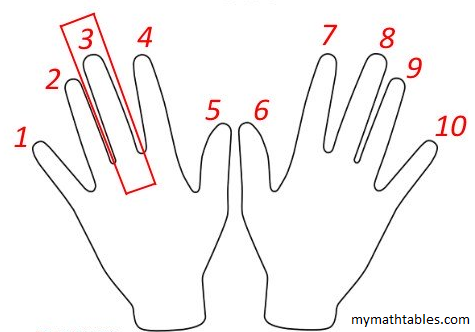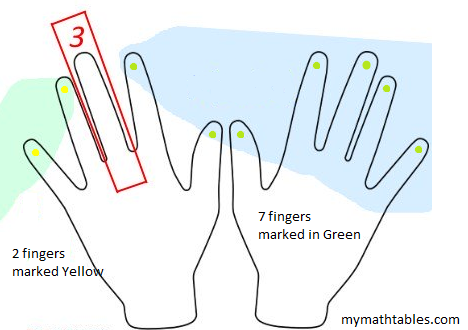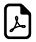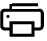# Nine Times Tables - Hand Fingers Trick# 9 Times Tables - How Fingers Trick Works

STEP1:Hold both your hands up with palms facing you. Number the fingers from left to right as one to ten.STEP2:Now hold down the finger of the number you want to multiply by 9. Here in this example, we are going to multiply by 3, so the 3rd finger is held down.

STEP3:The fingers to the left as the tens and the fingers to the right are units.

In this example, two to the left (marked yellow) and there are seven fingers to the right (marked green) which makes 27.So,the result is = 3 x 9 = 27# 9 Times Multiplication Table Finger Tricks

An online nine times table tricks.Easy way to learn 9 times table.# Nine Times Table Learning## How 9 times table works?

Think 9 times Table.Think Fingers !!!

#### What math times time do you want to generate ?###Times Table Tricks 2 to 11►

2 Times Table Trick

4 Times Table Trick

5 Times Table Trick

6 & 7 Times Table Trick

8 Times Table Trick

##Top Calculators ►

Tax Calculator, Calories Burned Calculator, PERT, SD Calculator, Dog Age Calculator, Children Equal Playing Time Calculator